## Example Questions

### Example Question #1 : X And Y Intercept

What is the y-intercept of the line that goes through the points (–2, 1) and (5, 6)?

67/7

–2/7

The answer cannot be determined from the given information.

17/7

0

17/7

Explanation:

The slope can be calculated from m = (y y1)/(x– x1) = (6 – 1)/(5 + 2). Having calculated the slope, we can now use point-slope form of a line, y – y= m(x – x1), and using the second point (5, 6): y – 6 = (5/7)(x – 5). This can be rearranged into slope-intercept form to obtain: y = (5/7)x + (17/7). Because the equation is now in slope intercept form, we know that the y-intercept is 17/7.

### Example Question #111 : Coordinate Geometry

Find the x-intercept of the equation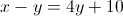–2

0

2

–10

10

10

Explanation:In order to find the x-intercept we simply let all the y's equal 0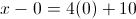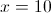### Example Question #1 : How To Find X Or Y Intercept

Quantity A:

The-intercept of the line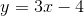Quantity B:

The-intercept of the line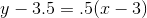The two quantities are equal.

Quantity B is greater

Quantity A is greater

The relationship cannot be determined from the information given.

The two quantities are equal.

Explanation:

The key to these quantitative comparison problems is to figure out the worth of both quantities, or figure out whether evaluating the quantities is even possible.  In this case, evaluating the quantities is a fairly straightforward case of figuring out the intercepts of two different lines, which is possible.  Therefore, you can already discount "the relationship cannot be determined from the information given".

To solve quantity A:is inform, whereis the-intercept. Therefore, the-intercept is equal toTo solve quantity B:, you have to sole for theintercept.  The quickest way to figure out the answer is to remember that theaxis exists at the line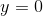, therefore to find out where the line crosses theaxis, you can setand solve for.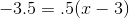-3.5 = .5x - 1.5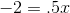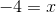Both quantity A and quantity B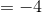, therefore the two quantities are equal.

### Example Question #1 : How To Find X Or Y Intercept

What is the-intercept of the following equation?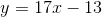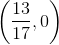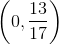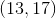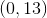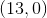Explanation:

To find the-intercept, you must plugin for.

This leaves you with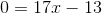.

Then you must get you by itself so you addto both sides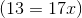.

Then divide both sides byto get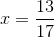.

For the coordinate point,goes first thenand the answer is.

### Example Question #111 : Coordinate Geometry

What is the slope of the line whose equation is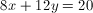?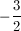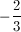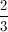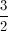Explanation:

Solve for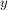so that the equation resembles the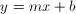form. This equation becomes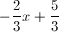. In this form, theis the slope, which is.

### Example Question #112 : Coordinate Geometry

Which of the following equations has a-intercept of?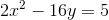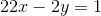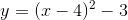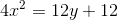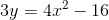Explanation:

To find the-intercept, you need to find the value of the equation where.  The easiest way to do this is to substitute infor your value ofand see where you getfor.  If you do this for each of your equations proposed as potential answers, you find thatis the answer.

Substitute infor: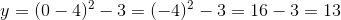### Example Question #7 : X And Y Intercept

Ifis a line that has a-intercept ofand an-intercept of, which of the following is the equation of a line that is perpendicular to?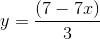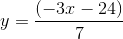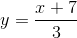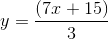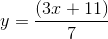Explanation:

Ifhas a-intercept of, then it must pass through the point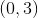.

If its-intercept is, then it must through the point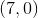.

The slope of this line is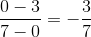.

Therefore, any line perpendicular to this line must have a slope equal to the negative reciprocal, which is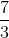. Onlyhas a slope of.

Tired of practice problems?

Try live online GRE prep today.